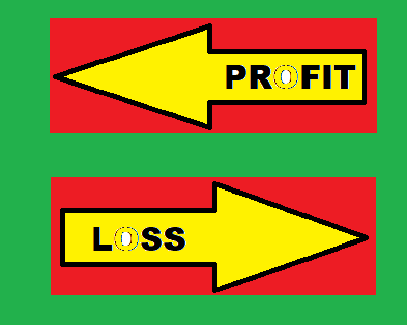# Profit and Loss Important Mathematics Formulas

Before learning Profit and Loss formulas you must know about some parameters like cost price, selling price, profit, and loss.Profit and Loss Important Mathematics Formulas

## Theory:

Cost Price (CP):    The money paid by the shopkeeper to the manufacturer or whole – seller to buy goods is called the cost price (cp) of the goods purchased by the shopkeeper.

Selling Price (SP): The price at which the shopkeeper sells the goods is called selling price (sp) of the goods sold by the shopkeeper to the customer.

Profit:                      If the selling price of an article is more than its cost price, then the dealer (or shopkeeper) makes a profit (or gain).

i.e., Profit = SP – CP; SP > CP

Loss:                       If the selling price of an article is less than its cost price, the dealer suffers a loss.

i.e., Loss = CP –SP; CP > SP

Some important formulae:

•               Profit = SP – CP
•             Loss = CP – SP
•                 Profit percentage = (Profit/CP)x 100
•                 Loss percentage =  (Loss/CP)x 100
•                S.P =  [(100 + Profit%)x CP]/100
OR

[(100 – Loss%) x CP]/100

•           C.P = (100 x SP)/ (100 + Profit%)
OR

(100 x SP)/ (100 – Loss%)
•           SP  = (100 + x)% of CP; when Profit = x% of CP
•                   SP =  (100 – x)% of CP; when Loss = x% of CP

Some other related Formulae:
•              Marked Price = CP + markup
•            Marked price = CP + (%marked x CP)/ 100
•               Selling price = Marked price – Discount
•          Selling price = MP - (%discount x MP)/ 100
Things to remember:

• If two items are sold at the same price, one with a profit of x% and another with a loss of x%, there will be an overall loss given by x2/100.
• If the CP of two items is the same, and one is sold at x% gain, other at x % loss, then the net loss or net profit=0.
• On an offer to buy “a” goods and get “b” goods free, i.e., if “a+b” articles are sold at the rate of “a” articles, then the percentage discount = [(b/(a+b))×100]
• If there are successive discounts of x% and y%, the effective discount can be calculated as [x+y – (xy/100) ]%
• There are many shopkeepers who cheat by using false weights, then the gain to the shopkeeper on using the false weight can be calculated as follows (100 + Gain%)/100=(True Weight)/(False Weight).

Also Read: